# 小白学 Python 数据分析（18）：Matplotlib（三）常用图表（上）

2019/04/10 10:10## 折线图

plot 函数的一般的调用形式如下：

#单条线：
plot([x], y, [fmt], *, data=None, **kwargs)

#多条线：
plot([x], y, [fmt], [x2], y2, [fmt2], ..., **kwargs)


plot(x, y, 'bo-')


plot(x, y, color='blue', marker='o', linestyle='-')


import matplotlib.pyplot as plt

help(plt.plot)


=============    ===============================
character        color
=============    ===============================
'b'          blue
'g'          green
'r'          red
'c'          cyan
'm'          magenta
'y'          yellow
'k'          black
'w'          white
=============    ===============================


=============    ===============================
character        description
=============    ===============================
'.'          point marker
','          pixel marker
'o'          circle marker
'v'          triangle_down marker
'^'          triangle_up marker
'<'          triangle_left marker
'>'          triangle_right marker
'1'          tri_down marker
'2'          tri_up marker
'3'          tri_left marker
'4'          tri_right marker
's'          square marker
'p'          pentagon marker
'*'          star marker
'h'          hexagon1 marker
'H'          hexagon2 marker
'+'          plus marker
'x'          x marker
'D'          diamond marker
'd'          thin_diamond marker
'|'          vline marker
'_'          hline marker
=============    ===============================


=============    ===============================
character        description
=============    ===============================
'-'          solid line style 实线
'--'         dashed line style 虚线
'-.'         dash-dot line style 点画线
':'          dotted line style 点线
=============    ===============================


import matplotlib.pyplot as plt

# 处理中文乱码
plt.rcParams['font.sans-serif']=['SimHei']

x_data = [2011,2012,2013,2014,2015,2016,2017]
y_data = [58000,60200,63000,71000,84000,90500,107000]
y_data_1 = [78000,80200,93000,101000,64000,70500,87000]

plt.title(label='xxx 公司 xxx 产品销量')
# 设置标题
plt.plot(x_data, y_data, linestyle = '-.', label = '产品销量')
plt.plot(x_data, y_data_1, label = '用户增长数')
# 开启网格线
plt.grid(True)
# 设置图例
plt.legend()
# 文件保存
plt.savefig("plot_demo.png")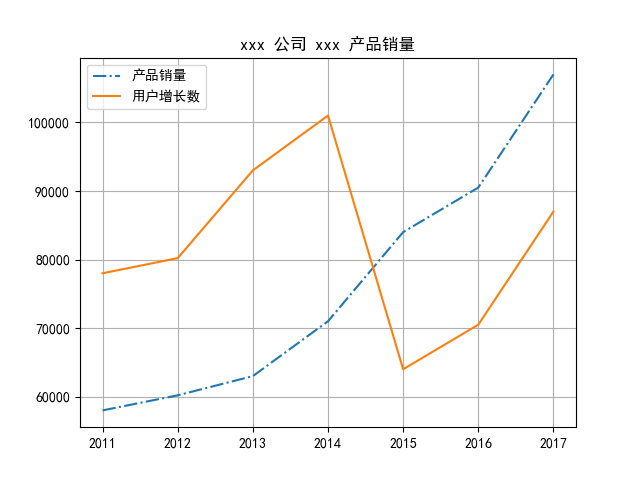## 柱状图

### 普通柱状图

atplotlib.pyplot.bar(left, height, width=0.8, bottom=None, hold=None, data=None, **kwargs)


left array x 轴；
height array 柱形图的高度，也就是y轴的数值；
alpha 数值 柱形图的颜色透明度 ； 1
width 数值 柱形图的宽度； 0.8
color（facecolor） string 柱形图填充的颜色； 随机色
edgecolor string 图形边缘颜色 None
label string 解释每个图像代表的含义
linewidth（linewidths / lw) 数值 边缘or线的宽度 1

import matplotlib.pyplot as plt
import numpy as np

# 处理中文乱码
plt.rcParams['font.sans-serif']=['SimHei']

x_data = np.array([2011,2012,2013,2014,2015,2016,2017])
y_data = np.array([58000,60200,63000,71000,84000,90500,107000])
y_data_1 = np.array([78000,80200,93000,101000,64000,70500,87000])

plt.title(label='xxx 公司 xxx 产品销量')

plt.bar(x_data, y_data, width=0.5, alpha=0.6, facecolor = 'deeppink', edgecolor = 'darkblue', lw=2, label='产品销量')

plt.legend()

plt.savefig("bar_demo_1.png")

• width : 柱子的宽度
• alpha : 透明度
• facecolor : 柱子填充色
• edgecolor : 柱子轮廓色
• lw : 柱子轮廓宽度
• label ： 图例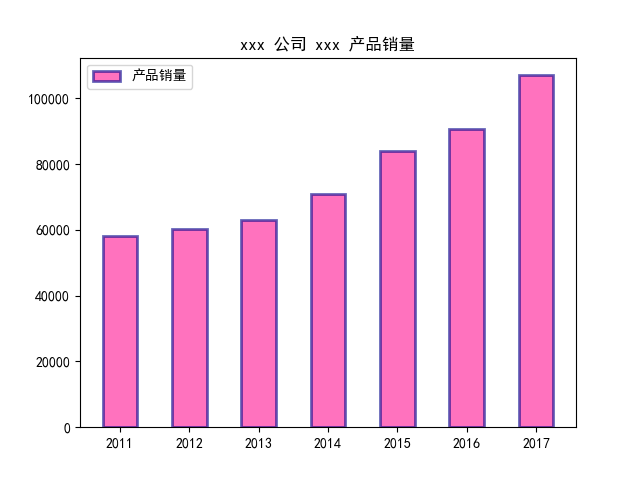### 并排柱状图

import matplotlib.pyplot as plt
import numpy as np

# 处理中文乱码
plt.rcParams['font.sans-serif']=['SimHei']

x_data = np.array([2011,2012,2013,2014,2015,2016,2017])
y_data = np.array([58000,60200,63000,71000,84000,90500,107000])
y_data_1 = np.array([78000,80200,93000,101000,64000,70500,87000])

plt.title(label='xxx 公司 xxx 产品销量')

plt.bar(x_data, y_data, width=0.3, alpha=0.6, facecolor = 'pink', edgecolor = 'blue', lw=1, label='产品销量')
plt.bar(x_data + 0.3, y_data_1, width=0.3, alpha=0.6, facecolor = 'blue', edgecolor = 'blue', lw=1, label='用户增长数')

plt.legend()

plt.savefig("bar_demo_2.png")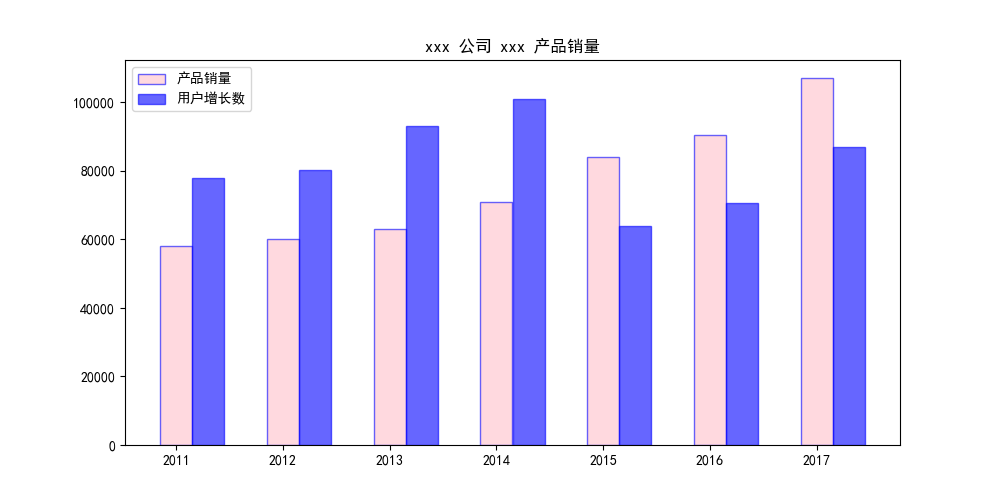### 堆积柱状图

import matplotlib.pyplot as plt
import numpy as np

# 处理中文乱码
plt.rcParams['font.sans-serif']=['SimHei']

x_data = np.array([2011,2012,2013,2014,2015,2016,2017])
y_data = np.array([58000,60200,63000,71000,84000,90500,107000])
y_data_1 = np.array([78000,80200,93000,101000,64000,70500,87000])

plt.title(label='xxx 公司 xxx 产品销量')

plt.bar(x_data, y_data, width=0.3, alpha=0.6, facecolor = 'pink', edgecolor = 'blue', lw=1, label='产品销量')
plt.bar(x_data, y_data_1, bottom=y_data, width=0.3, alpha=0.6, facecolor = 'blue', edgecolor = 'blue', lw=1, label='用户增长数')

plt.legend()

plt.savefig("bar_demo_3.png")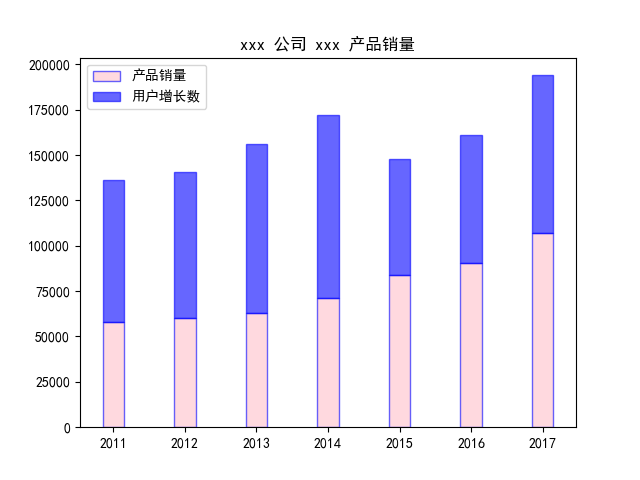### 横向柱状图

matplotlib.pyplot.barh(y, width, height=0.8, left=None, *, align='center', **kwargs)


import matplotlib.pyplot as plt
import numpy as np

# 处理中文乱码
plt.rcParams['font.sans-serif']=['SimHei']

x_data = np.array([2011,2012,2013,2014,2015,2016,2017])
y_data = np.array([58000,60200,63000,71000,84000,90500,107000])

plt.title(label='xxx 公司 xxx 产品销量')

plt.barh(x_data, y_data, alpha=0.6, facecolor = 'deeppink', edgecolor = 'deeppink', label='产品销量')

plt.legend()

plt.savefig("barh_demo.png")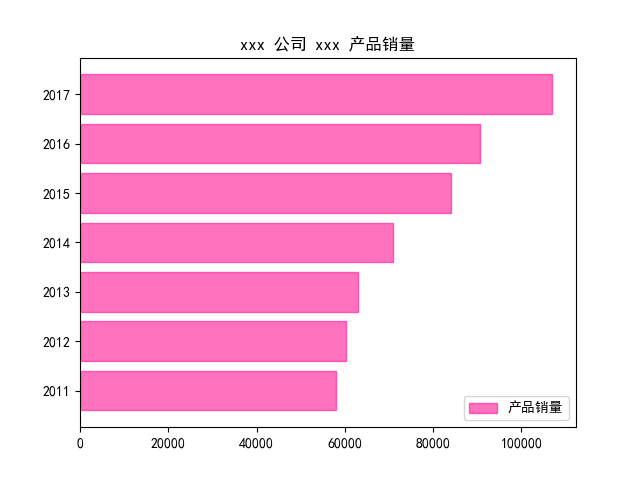## 参考

https://blog.csdn.net/sinat_36219858/article/details/79800460

https://blog.csdn.net/weixin_40683253/article/details/87641416### 作者的其它热门文章

0
0 收藏

0 评论
0 收藏
0